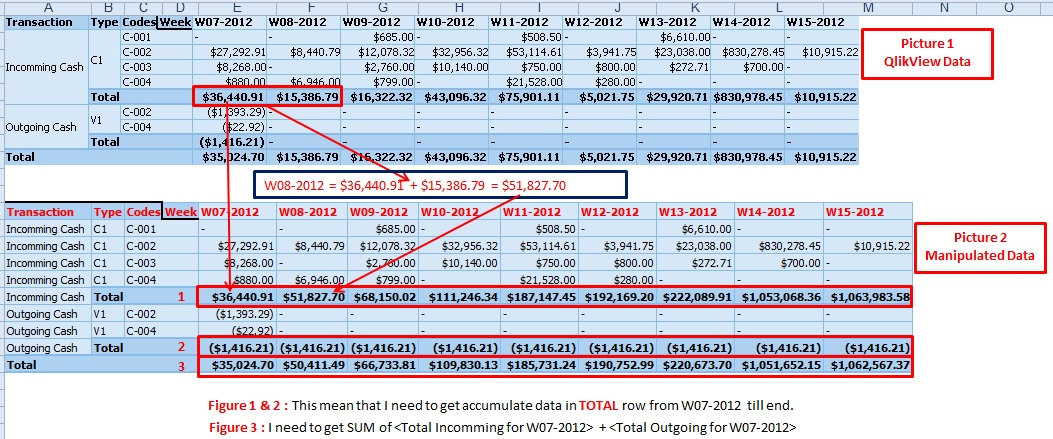# QlikView Layout & Visualizations

Discussion Board for collaboration on QlikView Layout & Visualizations.

Announcements
Coming your way, the Qlik Data Revolution Virtual Summit. October 27-29. REGISTER
cancel
Showing results for
Did you mean:
HighlightedMaster

## How to get Accumulate for Show Partial Sum in Pivot Table

Hi All,

I face one problem with Pivot Table for sum accumulate data. Let see the picture***For Pivot Table have

4 dimensions : Transaction, Type, Codes and Weeks

1 expression : SUM(Cash)

***The dimension <Weeks> is need to drag from vertical to horizontal

**For TOTAL in <Type> dimension

+ Incomming Cash

- Total in W07-2012 = \$36,440.91

- Total in W08-2012 = \$36,440.91 + \$15,386.79 = \$51,827.70

- Total in W09-2012 = \$36,440.91 + \$15,386.79 + \$16,322.32 = \$68,150.02

- And so on...

+ Outgoing Cash

- Total in W07-2012 = (\$1,416.21)

- Total in W08-2012 = (\$1,416.21) + 0 = (\$1,416.21)

- Total in W09-2012 = (\$1,416.21) + 0 + 0 = (\$1,416.21)

- And so on...

**For TOTAL in <Transaction> dimension

- Total in W07-2012 = \$36,440.91 + (\$1,416.21) = \$35,024.70

- Total in W08-2012 = \$51,827.70 + (\$1,416.21) = \$50,411.49

- Total in W09-2012 = \$68,150.02 + (\$1,416.21) = \$66,733.81

- And so on...

Enclosure

Pivot Cumm.qvw

Regards,

Sokkorn Cheav

Enclosure

Pivot Cumm.qvw

Regards,

Sokkorn Cheav

1 Solution

Accepted Solutions
HighlightedMVP & Luminary

Hi,

Try this expression.

=RangeSum(Before(TOTAL Sum(Cash), 0, ColumnNo(TOTAL)))

Regards,

Jagan.

5 Replies
HighlightedMaster

Hi,

Any one please me on this.

Regards,

Sokkorn Cheav

HighlightedMVP & Luminary

Hi,

I think it is not possible to change the Parital Sum alone, you can try this by using Above()/Before() methods but this would the individual values also along with Partial Sum.  You cannot manipulate the Partial sum values alone.

Regards,

Jagan.

HighlightedMaster

Hi Jagan,

Actually I get luck with: RangeSum(Above(TOTAL Sum(Cash), 0, RowNo(TOTAL)))

But this one working when the dimension <Weeks> is in vertical. Any idea if dimension <Weeks> is in horizontal?

Regards,

Sokkorn Cheav

HighlightedMVP & Luminary

Hi,

Try this expression.

=RangeSum(Before(TOTAL Sum(Cash), 0, ColumnNo(TOTAL)))

Regards,

Jagan.

HighlightedMaster

Hi Jagan,

Thanks once again for your help.

Regards,

Sokkorn Cheav